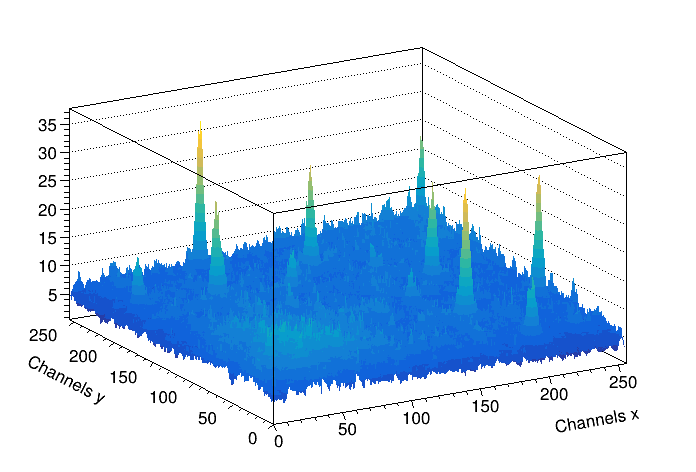# Smooth¶

Example to illustrate the Markov smoothing (class TSpectrum2).

Author: Miroslav Morhac, Olivier Couet
This notebook tutorial was automatically generated with ROOTBOOK-izer from the macro found in the ROOT repository on Sunday, October 02, 2022 at 09:38 AM.

In :
Int_t i, j;
const Int_t nbinsx = 256;
const Int_t nbinsy = 256;
Double_t xmin = 0;
Double_t xmax = (Double_t)nbinsx;
Double_t ymin = 0;
Double_t ymax = (Double_t)nbinsy;
Double_t** source = new Double_t*[nbinsx];
for (i=0;i<nbinsx;i++)
source[i] = new Double_t[nbinsy];
TString dir  = gROOT->GetTutorialDir();
TString file = dir+"/spectrum/TSpectrum2.root";
TFile *f     = new TFile(file.Data());
auto smooth = (TH2F*) f->Get("smooth1");
gStyle->SetOptStat(0);
auto *s = new TSpectrum2();
for (i = 0; i < nbinsx; i++){
for (j = 0; j < nbinsy; j++){
source[i][j] = smooth->GetBinContent(i + 1,j + 1);
}
}
s->SmoothMarkov(source,nbinsx,nbinsx,3); //5,7
for (i = 0; i < nbinsx; i++){
for (j = 0; j < nbinsy; j++)
smooth->SetBinContent(i + 1,j + 1, source[i][j]);
}
smooth->Draw("SURF2");

Info in <TCanvas::MakeDefCanvas>:  created default TCanvas with name c1


Draw all canvases

In :
gROOT->GetListOfCanvases()->Draw()